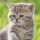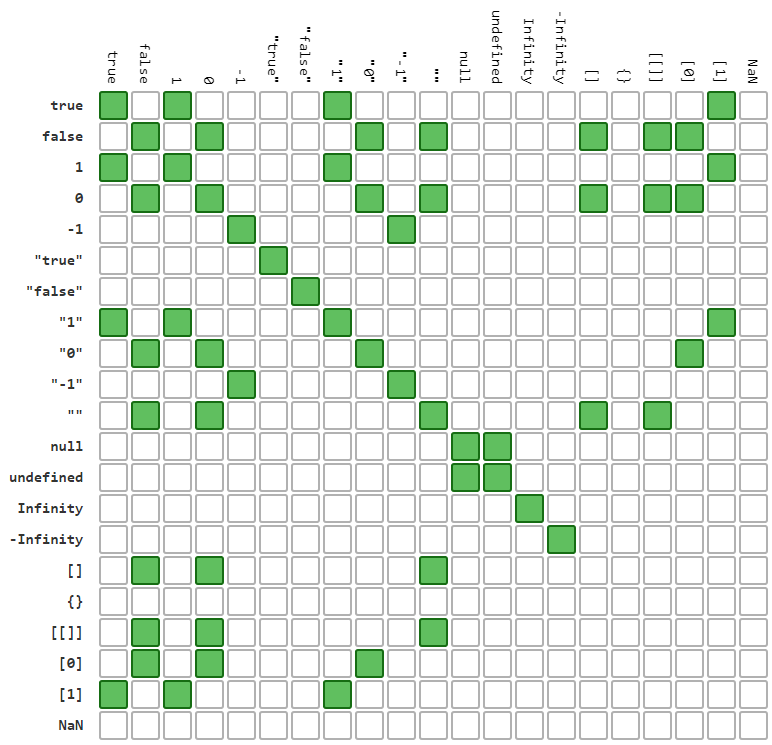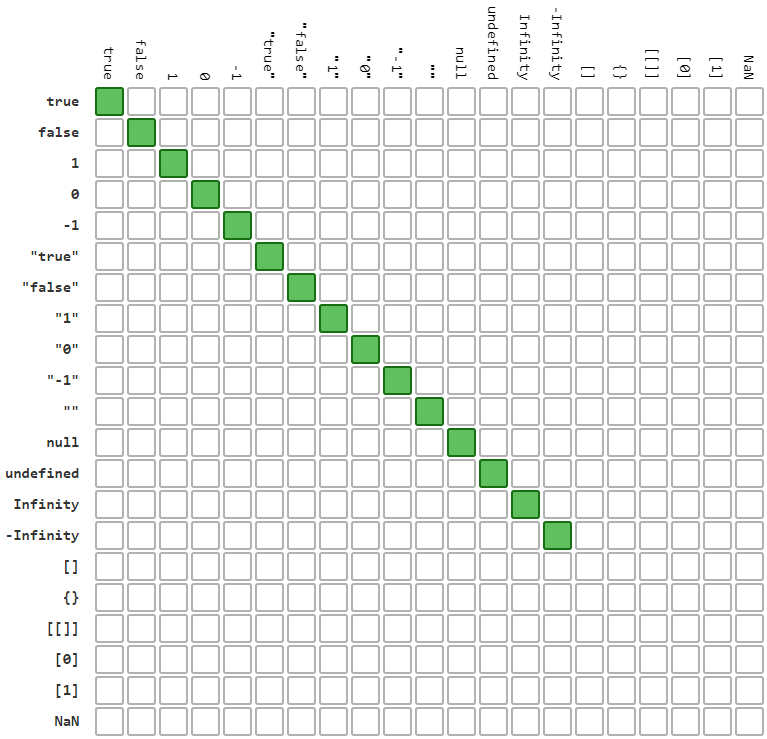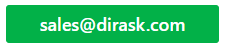Home
IT Knowledge
IT Career
Inspiration
Languages
EN

# JavaScript - what is the difference between == and === operators?

7 points
Created by:Kate_C
19800

In JavaScript there are available four different operators for checking two values are equal or not: ==, !=, === and !==. In this article we will try to describe difference beetwen them.

## 1. What is the difference between identity and equality operators (== vs ===) ?

Identity operator (===) works similar to equality operator (==) except variable type comparison - equality operator does not do it. Equality operator converts types if it is necessary and later chceck values - it manes to use this operator it is necessary to have knowledge about automatic types conversion because sometimes conversion result is not obvious. Identity operator starts with type comparision and later if they are same makes comparision.

## 2. Which operator should be used?

It is most common asked question.

Answert for this question is: Identity operator (===) should be used to avoid errors - it is JSLint suggestion too.

Simple case showing why identity operator should be used has been presented below:

``````// ONLINE-RUNNER:browser;

console.log( 25 ===  ); // false
console.log( 25  ==  ); // true``````

Note: in above example we can see the knowledge about used type is important - programmer making mistake ( - array with one element) can affect program will be working still correctly.

Solution: using identity operator.

## 3. JavaScript equality table example

Below material comes from GitHub.

Authtor put suggestion: Always use 3 equals unless you have a good reason to use 2.

### 3.1. `==` and `!=` comparison result

green means result for `==` is `true` (for `!=` `false`)

white means result for `!=` is `true` (for `==` `false`)

`==` operator result for different values / objects comparision:== operator result for different values / objects comparision - JavaScript

### 3.1. `===` and `!==` comparison result

green means result for `===` is `true` (for `!==` `false`)

white means result for `!==` is `true` (for `===` `false`)

`===` operator result for different values / objects comparision:=== operator result for different values / objects comparision - JavaScript